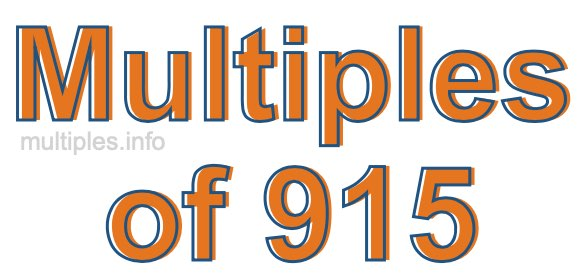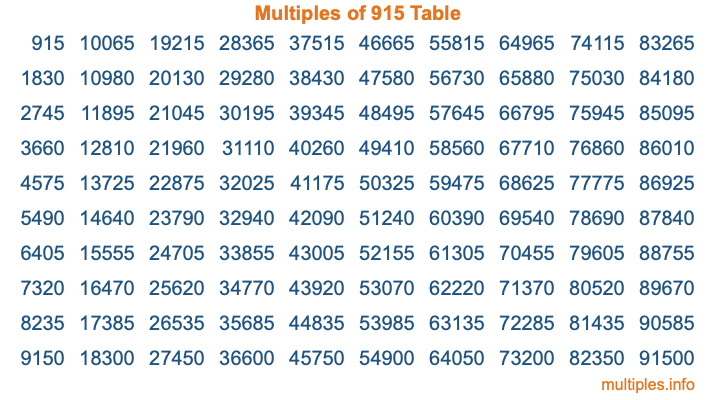Multiples of 915Welcome to the Multiples of 915 page. Here we will first teach you everything you will ever need to know about the multiples of 915, and then give you a study guide summary of everything we taught you to make sure you remember it all. Use this page to look up facts and learn information about the multiples of 915. This page will make you a multiples of nine hundred fifteen expert!

Definition of Multiples of 915
Multiples of 915 are all the numbers that when divided by 915 equal an integer. Each of the multiples of 915 are called a multiple. A multiple of 915 is created by multiplying 915 by an integer.

Therefore, to create a list of multiples of 915, you start with 1 multiplied by 915, then 2 multiplied by 915, then 3 multiplied by 915, and so on for as long as you want. Thus, the list of the first five multiples of 915 is 915, 1830, 2745, 3660, and 4575. To see a larger list of multiples of 915, see the printable image of Multiples of 915 further down on this page. We also have a category where you can choose any nth multiple of 915.

Multiples of 915 Checker
The Multiples of 915 Checker below checks to see if any number of your choice is a multiple of 915. In other words, it checks to see if there is any number (integer) that when multiplied by 915 will equal your number. To do that, we divide your number by 915. If the the quotient is an integer, then your number is a multiple of 915.

Is  a multiple of 915?

Least Common Multiple of 915 and ...
A Least Common Multiple (LCM) is the lowest multiple that two or more numbers have in common. This is also called the smallest common multiple or lowest common multiple and is useful to know when you are adding our subtracting fractions. Enter one or more numbers below (915 is already entered) to find the LCM.

Check out our LCM Calculator if you need more details about the Least Common Multiple or if you need the LCM for different numbers for adding and subtraction fractions.

nth Multiple of 915
As we stated above, 915 is the first multiple of 915, 1830 is the second multiple of 915, 2745 is the third multiple of 915, and so on. Enter a number below to find the nth multiple of 915.

th multiple of 915

Multiples of 915 vs Factors of 915
915 is a multiple of 915 and a factor of 915, but that is where the similarities end. All postive multiples of 915 are 915 or greater than 915. All positive factors of 915 are 915 or less than 915.

Below is the beginning list of multiples of 915 and the factors of 915 so you can compare:

Multiples of 915: 915, 1830, 2745, 3660, 4575, etc.

Factors of 915: 1, 3, 5, 15, 61, 183, 305, 915

As you can see, the multiples of 915 are all the numbers that you can divide by 915 to get a whole number. The factors of 915, on the other hand, are all the whole numbers that you can multiply by another whole number to get 915.

It's also interesting to note that if a number (x) is a factor of 915, then 915 will also be a multiple of that number (x).

Multiples of 915 vs Divisors of 915
The divisors of 915 are all the integers that 915 can be divided by evenly. Below is a list of the divisors of 915.

Divisors of 915: 1, 3, 5, 15, 61, 183, 305, 915

The interesting thing to note here is that if you take any multiple of 915 and divide it by a divisor of 915, you will see that the quotient is an integer.

Multiples of 915 Table
Below is an image of the first 100 multiples of 915 in a table. The table is in chronological order, column by column. The first column has the first ten multiples of 915, the second column has the next ten multiples of 915, and so on.The Multiples of 915 Table is also referred to as the 915 Times Table or Times Table of 915. You are welcome to print out our table for your studies.

Negative Multiples of 915
Although not often discussed or needed in math, it is worth mentioning that you can make a list of negative multiples of 915 by multiplying 915 by -1, then by -2, then by -3, and so on, to get the following list of negative multiples of 915:

-915, -1830, -2745, -3660, -4575, etc.

Multiples of 915 Summary
Below is a summary of important Multiples of 915 facts that we have discussed on this page. To retain the knowledge on this page, we recommend that you read through the summary and explain to yourself or a study partner why they hold true.

There are an infinite number of multiples of 915.

A multiple of 915 divided by 915 will equal a whole number.

915 divided by a factor of 915 equals a divisor of 915.

The nth multiple of 915 is n times 915.

The largest factor of 915 is equal to the first positive multiple of 915.

915 is a multiple of every factor of 915.

915 is a multiple of 915.

A multiple of 915 divided by a divisor of 915 equals an integer.

915 divided by a divisor of 915 equals a factor of 915.

Any integer times 915 will equal a multiple of 915.

Multiples of a Number
Here you can get the multiples of another number, all with the same attention to detail as we did for multiples of 915 on this page.

Multiples of
Multiples of 916
Did you find our page about multiples of nine hundred fifteen educational? Do you want more knowledge? Check out the multiples of the next number on our list!